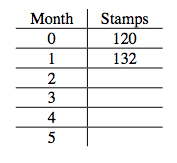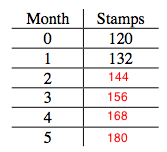### Home > AC > Chapter 14 > Lesson 14.2.1.3 > Problem2-39

2-39.

Lona received a stamp collection from her grandmother. The collection is in a leather book and currently has $120$ stamps. Lona joined a stamp club, which sends her $12$ new stamps each month. The stamp book holds a maximum of $500$ stamps.

1. Complete the table below.

Continue adding $12$ to find months 2-5.2. How many stamps will Lona have after one year?

See part (a). (Continue until month twelve.)

3. Write an equation to represent the total number of stamps that Lona has in her collection after n months. Let the total be represented by $t\left(n\right)$.

Remember that the initial amount was $120$ stamps and the rate is $12$ stamps per month.

Use these values to make an equation.

$t\left(n\right) = 120 +12n$

4. Solve your equation for $n$ when $t\left(n\right) = 500$. Will Lona be able to fill her book exactly with no stamps remaining? How do you know? When will the book be filled?

Month

Stamps

$0$

$120$

$1$

$132$

$2$

$3$

$4$

$5$

Substitute $500$ for $t\left(n\right)$.

$500 = 120 + 12n$

Solve for $n$.

$\textit{n}=31\frac{2}{3}$

Lona will not be able to fill her book exactly, with no leftover stamps, because when the equation is solved for $n$, the result is a mixed number. It will be filled in $32$ months.